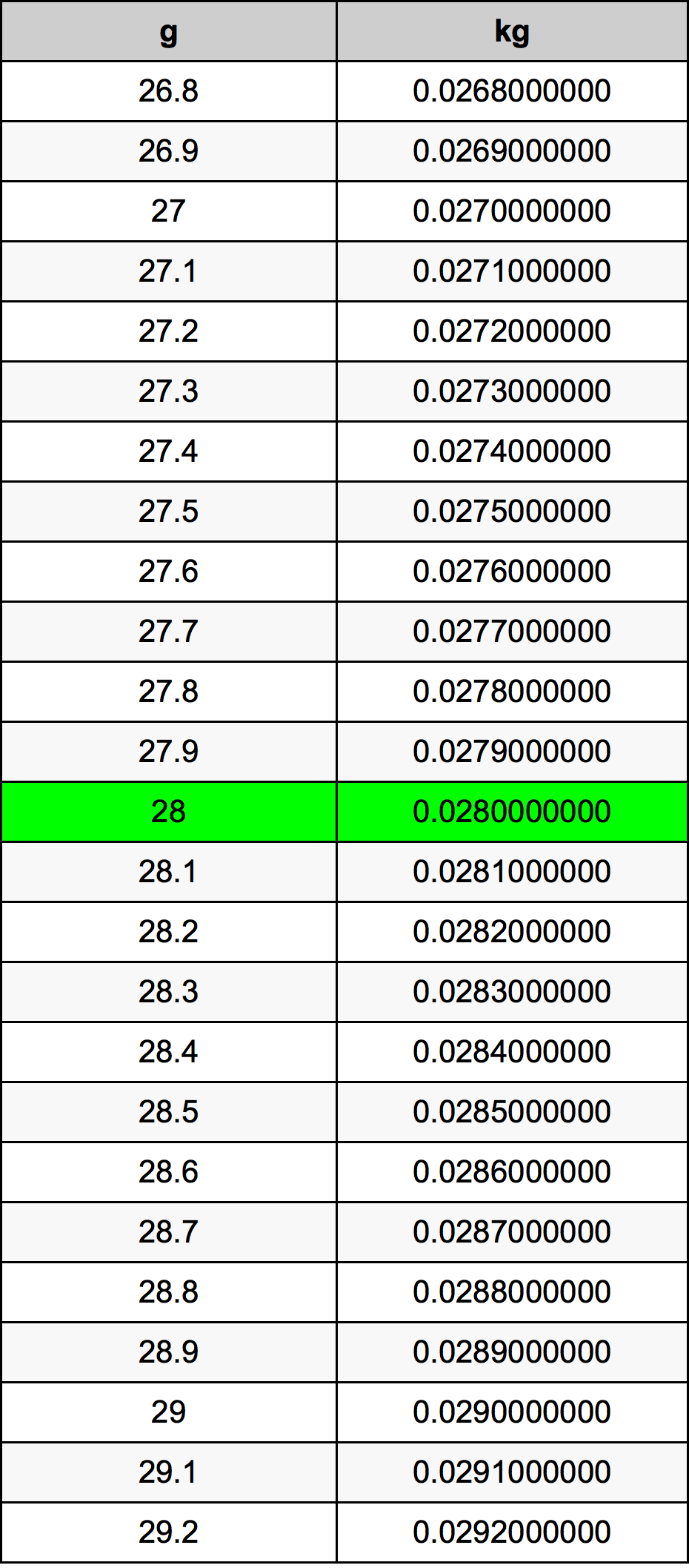Grams To Kilograms

# 28 g to kg28 Grams to Kilograms

g
=
kg

## How to convert 28 grams to kilograms?

 28 g * 0.001 kg = 0.028 kg 1 g
A common question is How many gram in 28 kilogram? And the answer is 28000.0 g in 28 kg. Likewise the question how many kilogram in 28 gram has the answer of 0.028 kg in 28 g.

## How much are 28 grams in kilograms?

28 grams equal 0.028 kilograms (28g = 0.028kg). Converting 28 g to kg is easy. Simply use our calculator above, or apply the formula to change the length 28 g to kg.

## Convert 28 g to common mass

UnitMass
Microgram28000000.0 µg
Milligram28000.0 mg
Gram28.0 g
Ounce0.9876709346 oz
Pound0.0617294334 lbs
Kilogram0.028 kg
Stone0.0044092452 st
US ton3.08647e-05 ton
Tonne2.8e-05 t
Imperial ton2.75578e-05 Long tons

## What is 28 grams in kg?

To convert 28 g to kg multiply the mass in grams by 0.001. The 28 g in kg formula is [kg] = 28 * 0.001. Thus, for 28 grams in kilogram we get 0.028 kg.

## 28 Gram Conversion Table## Alternative spelling

28 g to Kilograms, 28 g in Kilograms, 28 Gram to Kilograms, 28 Gram in Kilograms, 28 g to kg, 28 g in kg, 28 Gram to kg, 28 Gram in kg, 28 Grams to Kilograms, 28 Grams in Kilograms, 28 g to Kilogram, 28 g in Kilogram, 28 Grams to Kilogram, 28 Grams in Kilogram